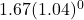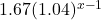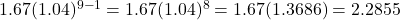Question

a plant grows by 1.67cm in its first week. each week it grows by 4% more than it did the week before. by how much will grow in nine weeks, including the first week?​

1.RuslanHeatt

Step-by-step explanation:

• Week 1 = 1.67 or• Week 2 = 1.67 + (1.67 · 4%) = 1.67 + 1.67 · 0.04 = 1.67(1 + 0.04) = 1.67(1.04)¹
• Week 3 = 1.67(1.04) + [1.67(1.04) · 0.04] = 1.67(1.04)(1.04) = 1.67(1.04)²
• Week 4 = 1.67(1.04)² + [1.67(1.04)² · 0.04] = 1.67(1.04)²(1.04) = 1.67(1.04)³

See a pattern?

• Number of week = x
• Plant growth =Week 9 =